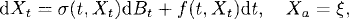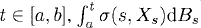# STOCHASTIC DIFFERENTIAL EQUATIONS

A stochastic differential equation is a differential equation that involves stochastic processes. The solution of a stochastic differential equation is also a stochastic process. In this chapter, we present stochastic differential equations and their solutions.

# 38.1 Basic Concepts and Facts

Definition 38.1 (Strong Solution of SDE). A stochastic process {Xt : atb} is called a strong solution of the following stochastic differential equation

(38.1)if it satisfies the following conditions:

(a) The stochastic process σ(t, Xt) belongs toad(Ω, L2[a, b]) so that for eachis an Itô integral.
(b) Almost all sample paths of f(t, Xt) belong to L1[a, b].
(c) For each t, the following equation

(38.2)holds almost surely.

Definition 38.2 (Weak Solution of SDE). If there exist a probability space with a filtration, a Brownian motion {t : t ≥ 0}, and a process {t : atb} adapted to the filtration such that they satisfy the conditions given in ...

Get Measure, Probability, and Mathematical Finance: A Problem-Oriented Approach now with the O’Reilly learning platform.

O’Reilly members experience live online training, plus books, videos, and digital content from nearly 200 publishers.